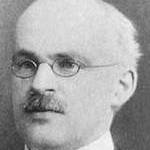# Mathematician of the dayThe above picture is taken from the following page:
http://www-history.mcs.st-and.ac.uk/Posters/1001.html

The complete list of the mathematician who were born or died today is given at the following URL

It is worth mentioning that “The MacTutor History of Mathematics archive” is a database of famous mathematician and mathematics archive created by by John J O'Connor and Edmund F Robertson is available at the following URL:

# List of Mathematician and facebook page links:

#### April 30: Carl Friedrich Gauss

Carl Friedrich Gauss was born on 30 April 1777. He worked in a wide variety of fields in both mathematics and physics including number theory, analysis, differential geometry, geodesy, magnetism, astronomy and optics. At the age of seven, Gauss summed the integers from 1 to 100 instantly by spotting that the sum was 50 pairs of numbers each pair summing to 101.

Quatation by Carl Friedrich Gauss: Mathematics is the queen of the sciences and number theory is the queen of mathematics.

• mathematician_of_the_day